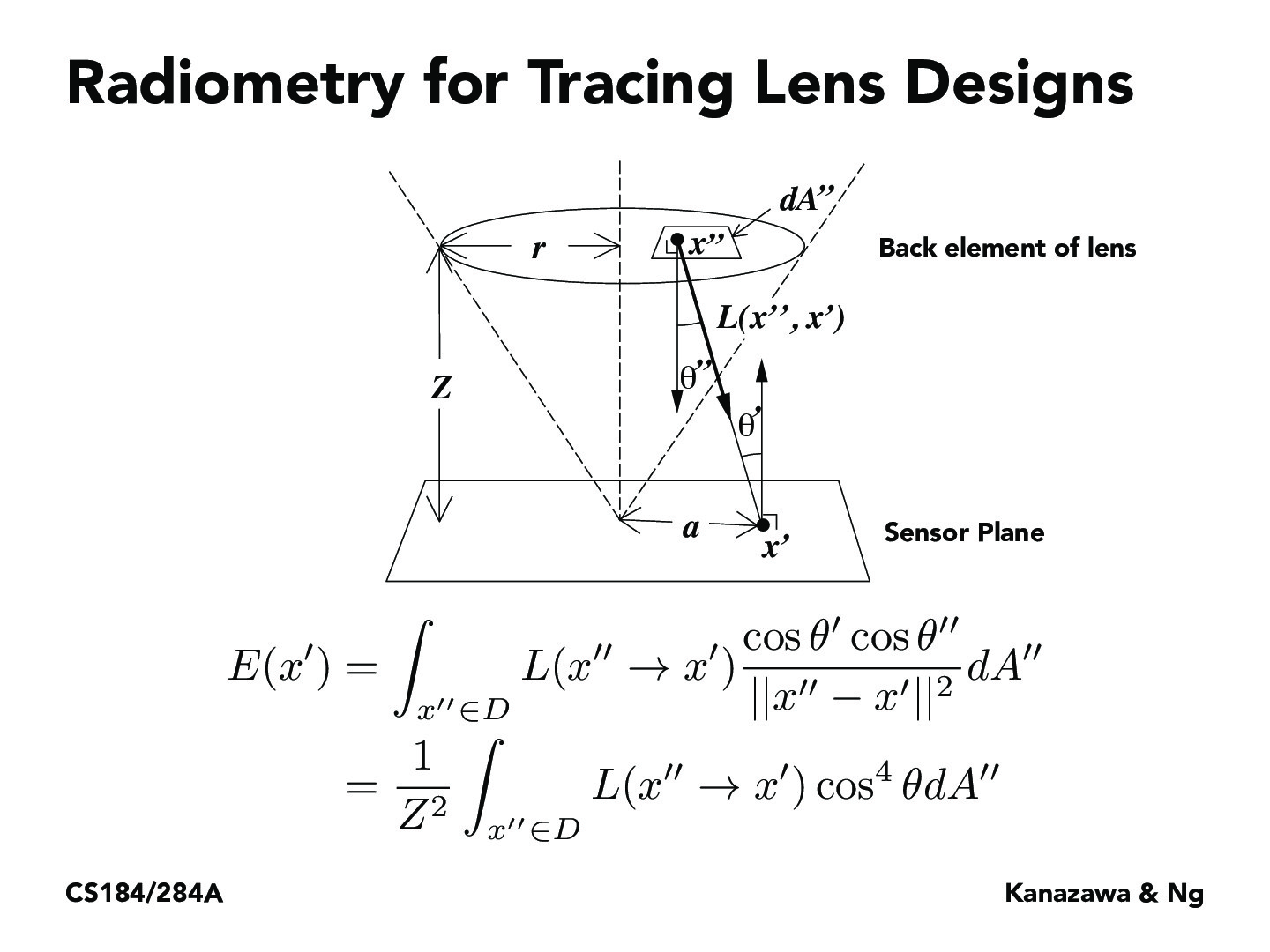Lecture 15: Cameras & Lenses (121)pixelled

Remember the relationship between irradiance and incident radiance is $E = \int_x L(x'' \rightarrow x')cos{\theta'} dw$. Based on the graph, $dw$ is $\frac{cos{\theta''}dA''}{(x'' - x1)^2}$. Replacing $dw$ with $dA''$, We have the equation in the slide.

You must be enrolled in the course to comment